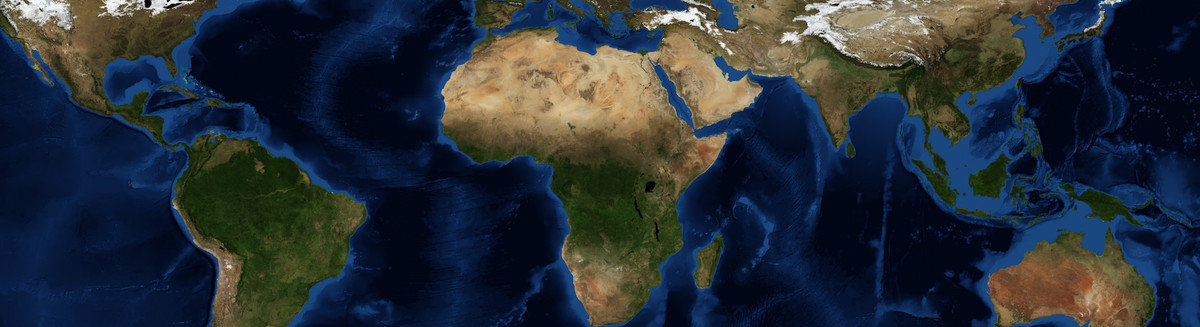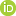This is a Preprint and has not been peer reviewed. The published version of this Preprint is available: https://doi.org/10.1016/j.epsl.2020.116202. This is version 4 of this Preprint.

##### Downloads

Download Preprint

##### Authors

Elias Rafn Heimisson##### Abstract

Faults in nature demonstrate fluctuations from planarity at most length scales that are relevant for earthquake dynamics. These fluctuations may influence all stages of the seismic cycle; earthquake nucleation, propagation, arrest, and inter-seismic behavior. Here I show quasi-dynamic plane-strain simulations of earthquake cycles on a self-similar and finite 10 km long rough fault with amplitude-to-wavelength ratio $\alpha = 0.01$. The minimum roughness wavelength, $\lambda_{min}$, and nucleation length scales are well resolved and much smaller than the fault length. Stress relaxation and fault loading is implemented using a variation of the backslip approach, which allows for efficient simulations of multiple cycles without stresses becoming unrealistically large. I explore varying $\lambda_{min}$ for the same stochastically generated realization of a rough fractal fault. Decreasing $\lambda_{min}$ causes the minimum and maximum earthquakes sizes to decrease. Thus the fault seismicity is characterized by smaller and more numerous earthquakes, on the other hand, increasing the $\lambda_{min}$ results in fewer and larger events. However, in all cases, the inferred b-value is constant and the same as for a reference no-roughness simulation ($\alpha = 0$). I identify a new mechanism for generating pulse-like ruptures. Seismic events are initially crack-like, but at a critical length scale, they continue to propagate as pulses, locking in an approximately fixed amount of slip. I investigate this transition using simple arguments and derive a characteristic pulse length, $L_c = {\lambda_{min}}/({4 \pi^4 \alpha^2})$ and slip distance, $\delta_c$ based on roughness drag. I hypothesize that the ratio $\lambda_{min}/\alpha^2$ can be roughly estimated from kinematic rupture models. Furthermore, I suggest that when the fault size is much larger than $L_c$, then most space-time characteristics of slip differ between a rough fault and a corresponding planar fault.

##### DOI

https://doi.org/10.31223/osf.io/7aubs

##### Subjects

Earth Sciences, Engineering, Geophysics and Seismology, Mechanical Engineering, Physical Sciences and Mathematics, Tectonics and Structure, Tribology

##### Keywords

earthquake, rate-and-state friction, Earthquake cycle simulations, Earthquake ruptures, Earthquake statistics, Pulses, Rough faults

##### Dates

Published: 2019-10-08 16:05

Last Updated: 2020-03-10 02:51

##### License

CC BY Attribution 4.0 International

Add a Comment

You must log in to post a comment.

Comments

There are no comments or no comments have been made public for this article.